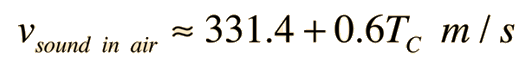# Speed of Sound in Air

The speed of sound in dry air is given approximately byfor temperatures reasonably close to room temperaature, where TC is the celsius temperature,

so that at temperature C = F,

the speed of sound is m/s = ft/s = mi/hr.

This calculation is usually accurate enough for dry air, but for great precision one must examine the more general relationship for sound speed in gases. If you measured sound speed in your oven, you would find that this relationship doesn't fit. At 200°C this relationship gives 453 m/s while the more accurate formula gives 436 m/s. This sound speed does not apply to gases other than air, for example the helium from a balloon.

It is important to note that the sound speed in air is determined by the air itself. It is not dependent upon the sound amplitude, frequency or wavelength.

Calculation note: You may enter temperature to calculate sound speed, or enter sound speed to calculate the corresponding temperature.

 Sound speeds in other gases Wave speeds in other media
 Relation to frequency and wavelength
 Breaking the sound barrier with an aircraft
Index

Traveling wave concepts

Sound propagation concepts

 HyperPhysics***** Sound R Nave
Go Back

# Selected Sound Speeds in Gases

 Gas Temperature (°C) Speed in m/s Air 0 331.5 Air 20 344 Hydrogen 0 1270 Carbon dioxide 0 258 Helium 20 927 Water vapor 35 402
 Sound speed in air Sound speed in helium
Index

Traveling wave concepts

Sound propagation concepts

Reference
Backus

 HyperPhysics***** Sound R Nave
Go Back

# Sound Speed in Helium

The speed of sound in helium at 0°C is about 972 m/s, compared to 331 m/s in air. This is consistent with the general relationship for sound speed in gases since the density of helium is so much less than that of air.

The high speed of sound is responsible for the amusing "Donald Duck" voice which occurs when someone has breathed in helium from a balloon. Note that if the vibration frequency of the vocal folds does not change, the actual pitch of the voice is not higher. The cavity resonances which determine the vocal formants would be raised by the higher sound speed, so the timbre of the voice would be different. It is possible for the pitch of the voice to change since gas dynamics ( i.e., Bernoulli effect) is partially responsible for the closing frequency of the vocal folds, but I haven't been able to find any data which demonstrates such a change.

Index

Traveling wave concepts

Sound propagation concepts

 HyperPhysics***** Sound R Nave
Go Back